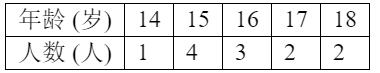$\text{A.}$ 15,16 $\text{B.}$ 15,15 $\text{C.}$ 15,15.5 $\text{D.}$ 16,15

A